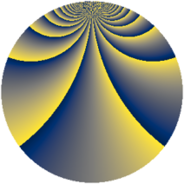# Properties

 Label 1520.2.emLevel $1520$ Weight $2$ Character orbit 1520.em Rep. character $\chi_{1520}(61,\cdot)$ Character field $\Q(\zeta_{36})$ Dimension $1920$ Sturm bound $480$

# Related objects

## Defining parameters

 Level: $$N$$ $$=$$ $$1520 = 2^{4} \cdot 5 \cdot 19$$ Weight: $$k$$ $$=$$ $$2$$ Character orbit: $$[\chi]$$ $$=$$ 1520.em (of order $$36$$ and degree $$12$$) Character conductor: $$\operatorname{cond}(\chi)$$ $$=$$ $$304$$ Character field: $$\Q(\zeta_{36})$$ Sturm bound: $$480$$

## Dimensions

The following table gives the dimensions of various subspaces of $$M_{2}(1520, [\chi])$$.

Total New Old
Modular forms 2928 1920 1008
Cusp forms 2832 1920 912
Eisenstein series 96 0 96

## Trace form

 $$1920q + O(q^{10})$$ $$1920q - 12q^{10} + 12q^{16} + 48q^{26} + 120q^{34} + 48q^{36} - 60q^{38} + 240q^{42} - 132q^{44} - 60q^{46} + 960q^{49} - 24q^{51} - 96q^{52} - 180q^{54} + 120q^{66} - 72q^{68} + 48q^{69} + 168q^{72} + 168q^{74} + 96q^{76} - 96q^{85} - 108q^{90} - 312q^{96} - 84q^{98} + O(q^{100})$$

## Decomposition of $$S_{2}^{\mathrm{new}}(1520, [\chi])$$ into newform subspaces

The newforms in this space have not yet been added to the LMFDB.

## Decomposition of $$S_{2}^{\mathrm{old}}(1520, [\chi])$$ into lower level spaces

$$S_{2}^{\mathrm{old}}(1520, [\chi]) \cong$$ $$S_{2}^{\mathrm{new}}(304, [\chi])$$$$^{\oplus 2}$$